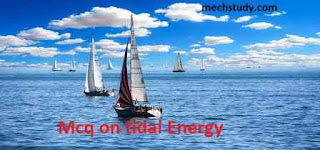Mcq on tidal energy (objectives)

Hello guys we know that wind energy is one of Renewable energy resources. this  lession  today we solve Renewable energy subjects Multiple choice questions Mcq on tidal energy (objectives)

Guys this tidal energy mcq is important for all competitive exam.

Know without wasting time let's begin to start tidal energy mcqs ( objectives) questions with answers

Mcq on tidal energy (objectives)Mcq on tidal energy (objectives)

Know start mcq

1. Tidal energy utilizes

A. Kinetic energy of water

B. Potential energy of water

C. Both A and B

D. None of these

Ans - A

2. In ocean tides are genrate due to.....

A. Sun

B. Moon

C. Both A and B

D. None of these

Ans - C

3. Tidal energy is a ..........

A. Renewable energy

B. Non Renewable energy

C. Commercial energy

D. None of these

Ans - A

4. In which country has worlds largest (bigger) tidal power plant?

A. Netherland

B. Australia

C. South korea

D. India

Ans - C

5. What is full form of OTEC ?

A. Ocean thermal energy consumption

B. Ocean techno energy conservation

C. Ocean thermal energy cultivation

D. Ocean thermal energy conservation

Ans - D

6. How is OTEC caused

A. By Geothermal energy

B. By solar energy

C. By gravitation force

D. By wind energy

Ans - B

7. How many high peaks occurs in a single pool tidal system?

A. One

B. Two

C. Three

D. Four

Ans - B

8. What is a tidal mill ?

A. A water mill drive by tidal rise and fall.

B. A dam to store the tide

C. A lagoon

D. A barrage

Ans - A

9. Strong coast parallel oscillating tidal concurrent are found in ..............

A. China

B. India

C. USA

D. Japan

Ans - A

10. What is the difference between water hight at low tides and water height at high tide.......

A. Tidal volume

B. Tidal variation

C. Tidal current

D. Tidal range

Ans - D

11. Construction cost of tidal power plant is ........

A. High

B. Low

Ans - A

13. Construction time required for tidal power plant is ........

A. High

B. Low

Ans - A

14. How many low tides occurring in each day ?

A. One

B. Two

C. Three

D. Four

Ans - B

15. Bulges of water are called..........

A. Low tide

B  high tide

C. None of these

Ans - A

16. Wher is  potential for developing tidal power plant in india is in ..........

A. San Jose

B. Kutch

C. Ran

D. Severn

Ans - B

17. Tidal are rhythmic and constant.

A. True

B. False

Ans - B

Solution- tidal are rhythmic but not constant is always very.

18. In tidal energy power plant water to the turbine is allowed through the.......

A. Pump

B. Pipe

C. Sluice gates

D. Canals

Ans - B

19. Which types of turbine generally used in tidal energy power plant

A. Pelton wheel

B. Francis turbine.

C. Gorlov helical turbine

D. Kaplan turbine

Ans - D

20. Tidal energy power plant is very high costly and it requires large cross section area.

A. true

B. false

Ans - A

In this  lession  today we solve Renewable energy subjects Multiple choice questions Mcq on tidal energy (objectives)

If you have any questions about wind energy  then comment below.

Previous
Next Post »